Articles » Science and Technology » Concept » The Simple Concept of Expectation – maximization (EM) Algorithm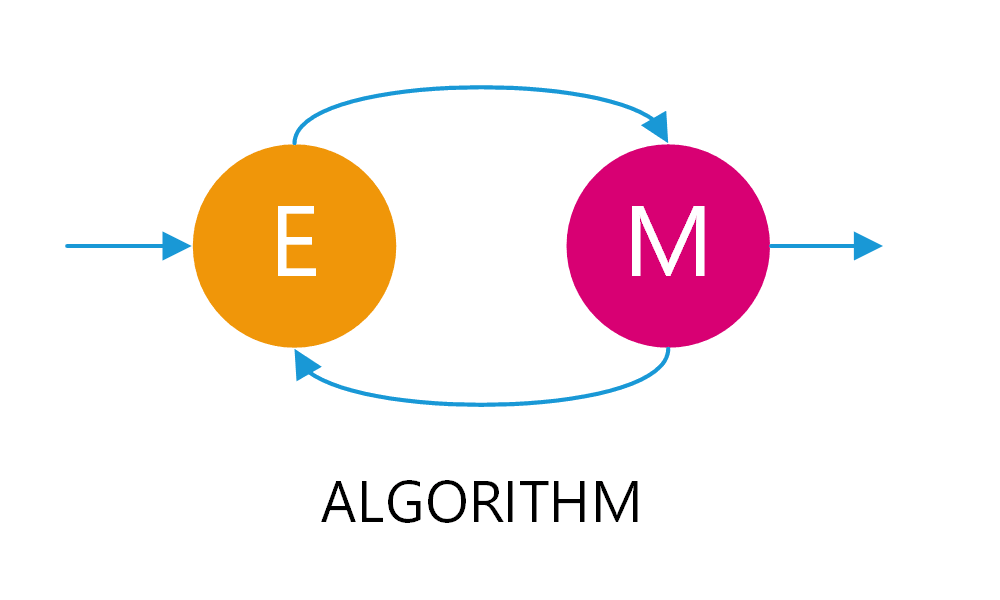# The Simple Concept of Expectation – maximization (EM) Algorithm

Last Updated on May 27, 2022

Learn an algorithm in autodidact is time-consuming; especially for people who are not interested in mathematics. Because, sometimes we don’t know that “we don’t know”, many things. Including when studying the Expectation – maximization (EM) Algorithm. Therefore, I could improve your understanding of the EM algorithm in this article, and I hope you can easily understand it.

EM algorithm often used in machine learning as an algorithm for data clustering.​, ​ Sometimes, one of the clustering problems is when the data doesn’t have any labels. For example, if we know A in the data, then we can calculate B from the data; or vice versa, if we know B, we can calculate A. However, sometimes we have mixed data, and the label of A and B are unknown. In this case, the EM algorithm could help to solve the problem.​​

For a summary, the EM algorithm is an iterative method, involves expectation (E-step) and maximization (M-step); to find the local maximum likelihood from the data. Commonly, EM used on several distributions or statistical models, where there are one or more unknown variables. Therefore, it called missing or “latent” variables.

In other words, we can use the EM algorithm if:

• We have incomplete datasets (for example, it doesn’t have groups or labels in the data), but we need to predict its group or label.
• The group or label has never been observed or recorded but is very important in explaining the data. It called a “latent” variable, meaning missing, hidden, or invisible.

Note: Some theories that you may need to learn are: the probability of binomial random variable and conditional probability.

## Case example for EM Algorithm

To easily understand the EM Algorithm, we can use an example of the coin tosses distribution.​​

For example, I have 2 coins; Coin A and Coin B; where both have a different head-up probability. I will randomly choose a coin 5 times, whether coin A or B. Then, each coin selection is followed by tossing it 10 times. Therefore, we have the following outcomes:

• Set 1: H T T T H H T H T H (5H 5T)
• Set 2: H H H H T H H H H H (9H 1T)
• Set 3: H T H H H H H T H H (8H 2T)
• Set 4: H T H T T T H H T T (4H 6T)
• Set 5: T H H H T H H H T H (7H 3T)

From the fig. 1 above, the “image” side denoted by “H” or Head-up, and the “number” side denoted by “T” or Tail-up. Then, the probability of coin will land with head-up for each of these coins, could be denoted as theta or “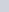“.

The question is, how do you estimatefor each coin?​​

## Completed data

In different cases, with the same outcomes as above; I give you more information. I told you which coins I used for each set; means the coin identity. Then, the data you have will be like this:

• Set 1, Coin B: H T T T H H T H T H (5H 5T)
• Set 2, Coin A: H H H H T H H H H H (9H 1T)
• Set 3, Coin A: H T H H H H H T H H (8H 2T)
• Set 4, Coin B: H T H T T T H H T T (4H 6T)
• Set 5, Coin A: T H H H T H H H T H (7H 3T)

Because you already have all the data you need, you can easily calculate the probability of getting head-up on each coin. Therefore, the calculation ofwill be:

By knowing the result of coin A = 24H 6T, in 3 sets with a total of 30 tosses, then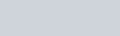.

Similarly to the result of coin B = 9H 11T, in 2 sets with a total of 20 tosses, then.

This simple equation is known as Maximum Likelihood Estimation (MLE), Where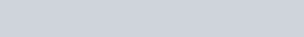.

## The overview of EM algorithm

### Overall flowchart

Before returning to the original case, let me explain briefly the stages of the EM algorithm. So you have an overall picture of the EM algorithm flow, which helps you reading until the end.

There are several steps in the EM algorithm, which are:

1. Defining latent variables
2. Initial guessing
3. E-Step
4. M-Step
5. Stopping condition and the final result

Actually, the main point of EM is the iteration between E-step and M-step, which could be seen in Fig. 2 above. The E-step will estimate your hidden variables, and the M-step will re-update the parameters, based on the estimation of the hidden variables. In other words, this iteration aims to re-improve the estimation of current parameters.

### Inside the EM algorithm

Take a look at Fig 3 above, to see what EM really does in your case; where you don’t know the coin identity. The current approach is to distribute the number of heads for each set; proportionally based on theparameter for each coin. Therefore, first, you need to guess the initialparameter [Fig 3(1)] for each coin. Then, (in the beginning) the currentparameters [Fig 3(2)] are your guessing.

Next, in the E-step, you could calculate each coin’s probability to get the outcomes of each set [Fig 3(3)]; based on the currentparameters. For example, the probability of coin A to get 8H 2T in 10 tosses (set 3).

Then, compare the probabilities both coin A and coin B; based on their total probability [Fig 3(4)]. For example, the result for a set with 8H 2T, I have a 73% probability using coin A, compared to 27% using coin B. This is how you estimate the probability of coin identity used in each set.

Then, the estimated identity is used to estimate the number of heads (and tail) for each coin, for each set [Fig 3(5)]. For example, those 8 Heads divided proportionally 73% to coin A and 27% for coin B.

Finally, the estimation of total heads of each coin is used to recalculate the value of theparameters at the M-Step. This re-calculation will improve the current[Fig 3(2)]. In other words, this re-calculation is based on the estimated results of hidden variables and observable data.

This iteration will continue until reaches the “maximum iteration” limit or convergence threshold reached. When the iteration stopped, the final results obtained are the closest estimation to the local optimum solutions.

## 1. Defining latent variables

Now, back to the original case, where the outcomes you have are:

• Set 1: H T T T H H T H T H (5H 5T)
• Set 2: H H H H T H H H H H (9H 1T)
• Set 3: H T H H H H H T H H (8H 2T)
• Set 4: H T H T T T H H T T (4H 6T)
• Set 5: T H H H T H H H T H (7H 3T)

First, you must define what variables are required; but are not observed in the data.

The goal is to estimate the probability of getting heads-up for each coin. However, it cannot be calculated directly; if you don’t know the identity of the coin used in each set. Therefore, it is necessary to know which coin is used in each set. In other words, this coin identity is your latent variable.

Right now, besides the 5 sets of outcomes above, you only know that I used two coins; which are coin A and coin B.

## 2. Initial guessing

As stated before, the probability of getting head-up for each of these coins denoted as theta or. Currently, there are only coin A and coin B; with unknown parameter values of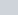and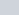.

Then, before estimating with EM iteration, you need to provide an initial estimate to both parameters [Fig 3(1)]. Therefore, you may choose randomly for each. For example, you may setis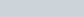, or else, it’s up to you; as well as.

By the way, please noted that there is no relationship between parameters guessing; bothand. For example, if you think that the sum ofandmust be = 1, NO!. This probability represents the individual value of getting heads-up on each coin.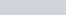, meaning that there is 60% probability of getting head-up, compared to 40% probability of getting tail-up using coin A. It has nothing to do with guessing the value ofor for coin B. So even though you already set the initial of, you may setwith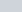,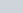,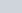,or any number you want between 0 and 1.

Okay enough. Now, let’s say you have randomly set both initial values, which are:and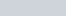## 3. E-Step

Now you have the required variables. So, you can start estimating the identity of the coin used in each set.

Each set, which contains the outcomes of heads and tails, can be denoted by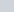notation. Then, the probability of “using coin A” denoted as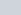, and the probability of “using coin B” denoted as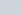.

### The probability a coin giving E

At the beginning of E-Step, you need to know the probability of a set using coins A or B. Take an example from set 3, where= HTHHHHHTHH (8H 2T). If, it means the probability of getting head is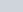(and tail) uses coin A. Then, how much probability of coin A will give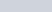in 10 tosses (a set)? This is what we need to estimate first [Fig 3(3)]. Then, this probability can be denoted by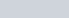.

Similarly, the probability of coin B giving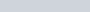, could be denoted by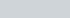. This actually relates to the probability distribution of a binomial random variable, which equation is: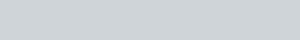where: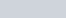= the probability of coins x gives.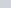= total coin tosses in a set.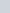= total number of heads in a set of.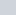= the probability of getting head-up using coin x.

Then, we can calculate the probability of gettingusing coins A and B as follows: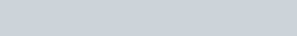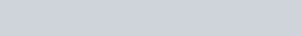### The ratio of coins A and B gives E

Next, you can compare the probabilities both of coin A and coin B give[Fig 3(4)]. So, according to Bayes’ theorem and the law of total probability, we can determine the ratio of probability using the following equation:​​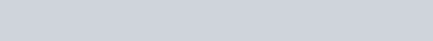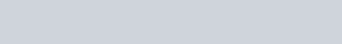where:= the probability of coin x giving(compared to coin y).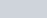= the probability of choosing coin x.= the probability of choosing coin y.

However, because our case is only using 2 coins, coins A and B, then the probability that I will choose one of them is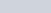. Then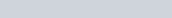.

Accordingly, for coin A, we can simplify the equation above into:Similarly, for coin B, become:### Estimates the number of heads for each coin

Previously, the ratio of coins A and B in giving eachneeds to be calculated. In this case, it is calculated from set 1 to 5. Then the results could be seen in the following table.

Coin TossesECoin A ProbabilityCoin B Probability
HTTTHHTHTH5H 5T0.450.55
HHHHTHHHHH9H 1T0.800.20
HTHHHHHTHH8H 2T0.730.27
HTHTTTHHTT4H 6T0.350.27
THHHTHHHTH7H 3T0.650.35

After that, you need to estimate the total number of H for each coin [Fig 3(5)]. It is calculated based on the coin ratio above. To calculate “total heads and tails” for coin x, it is similar to the “complete data”. The method is quite easy, you just need to multiply the ratio of each coin to the number of heads in each, the results are shown in the following table.

Coin TossesEEstimated H for Coin AEstimated H for Coin B
HTTTHHTHTH5H 5T5 * 0.45 = 2.255 * 0.55 = 2.75
HHHHTHHHHH9H 1T9 * 0.80 = 7.29 * 0.20 = 1.8
HTHHHHHTHH8H 2T8 * 0.73 = 5.848 * 0.27 = 2.16
HTHTTTHHTT4H 6T4 * 0.35 = 1.44 * 0.65 = 2.6
THHHTHHHTH7H 3T7 * 0.65 = 4.557 * 0.35 = 2.45

If you want, you can also calculate the tails for each coin as in the table above. But that is not necessary, because you can use another straightforward approach.

Until this step, you already have the E-Step calculations. Just a little bit more effort to finish your calculation in the M-Step.

## 4. M-Step

The results from the E-step can be used to improve theparameter. Here, we can use the maximum likelihood estimation (MLE) equation similar to the “completed data”.

As I said before, that it’s not necessary to calculate the tails for each coin; then you sum the heads and tails for each coin. Because each set contains 10 tosses, you just need to multiply the coin ratio with 10. That way, you could get the total estimated tosses from. Therefore:Finally, the both parameter ofandfor the first iteration have been improved [Fig 3(2)]. For the next iteration, the E-Step will use this new parameter value; and re-improved at the next M-step. This iteration will always repeat the E-step and M-step, until it reaches any stop condition.

## 5. Stopping condition and the final result

The iteration of the E-Step and M-Step, will be repeated until they meet the stopping condition. Commonly, the EM algorithm has two options of stopping condition, which are:

• Maximum iteration: means that, the EM Algorithm will stop if a certain number of iterations has been reached. For example, the maximum iteration is set to 10 iterations, then the EM Algorithm will not be more than 10 iterations. Or,
• Convergence threshold: means that, the M-step gives no significant parameter improvement; compared to the improvement in the previous iteration. The changes are very small below our threshold.

### The final result of EM algorithm

In the EM case example above, the parameter improvement in each iteration can be seen in the following table:

IterationθAθBDifferences
00.60.50.7810
10.7130.5810.1390
20.7450.5690.0342
30.7680.5500.0298
40.7830.5350.0212
50.7910.5260.0120
60.7950.5220.0057
70.7960.5210.0014
80.7960.5200.0010
90.7960.5200.0000

To calculate the differences or improvements in each iteration, you can use the Euclidean Distance equation, which is: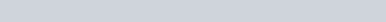In your case, an examples from iteration 1 to iteration 2 is:The final results will be taken, if one of the stop condition has been reached. It doesn’t matter which one is the first reached. For example, let’s just say that the maximum iteration limit is set to 10 iterations. It has been seen that the iteration above has been done 10 iterations (starting iterations from 0 to 9). However, if the Convergence Threshold set to a minimum of 0.001, then the iteration will stop at the 8th iteration. It because the convergence limit has been reached first, before the maximum iteration.

So in our case, in 8th iteration, the final result obtained for coin A is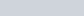, and for coin B is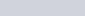.

## Weaknesses of the EM algorithm

Every algorithm has weaknesses, without any doubt; as well as the EM algorithm.

Every iteration in the EM algorithm, in general, will always improve the parameter closer to the local maximum likelihood. In other words, the EM algorithm will guarantee convergence, but will not guarantee to give a global maximum likelihood. And also there is no guarantee that you will get the maximum likelihood estimation (MLE).

In some conditions, the EM algorithm may give unexpected results. for example, if we set the initial parameter with the same number,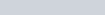.​​ Then the results are:

IterationθAθBDiff
00.30.30.7071
10.6660.6660.2263
20.6660.6660
30.6660.6660
40.6660.6660

It appears that there are no parameter improvements. For more details, try looking at Fig 4. “Case 1” is the normal case in the previous chapters, and “Case 2” is the case where we use the same initial parameter values.